JEE  >  Solved Examples - Solutions (Part - 4)

# Solved Examples - Solutions (Part - 4) Notes | Study Additional Documents and Tests for JEE - JEE

## Document Description: Solved Examples - Solutions (Part - 4) for JEE 2022 is part of Additional Documents and Tests for JEE preparation. The notes and questions for Solved Examples - Solutions (Part - 4) have been prepared according to the JEE exam syllabus. Information about Solved Examples - Solutions (Part - 4) covers topics like and Solved Examples - Solutions (Part - 4) Example, for JEE 2022 Exam. Find important definitions, questions, notes, meanings, examples, exercises and tests below for Solved Examples - Solutions (Part - 4).

Introduction of Solved Examples - Solutions (Part - 4) in English is available as part of our Additional Documents and Tests for JEE for JEE & Solved Examples - Solutions (Part - 4) in Hindi for Additional Documents and Tests for JEE course. Download more important topics related with notes, lectures and mock test series for JEE Exam by signing up for free. JEE: Solved Examples - Solutions (Part - 4) Notes | Study Additional Documents and Tests for JEE - JEE
 1 Crore+ students have signed up on EduRev. Have you?

Example 45. Sea water is 3.5% by mass of a salt and has a density 1.04 g cm-3 at 293 K. Assuming the salt to be sodium chloride, calculate the osmotic pressure of sea water. Assume complete ionization of the salt.
Solution. Mass of NaCl = 3.5 g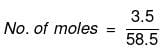Actual number of moles of particles of solute in solution=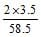Volume of solution = 1 litre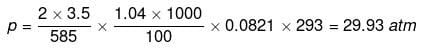Example 46. Molality of a solution in aqueous medium is 0.8. Calculate its mole fraction and the percentage by mass of solute if molar mass of solute is 60.
Solution. We know that,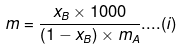where, x= mole fraction of solute
mA = molar mass of solvent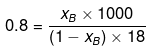xB = 0.014
Let wB = x g, wA = 100 g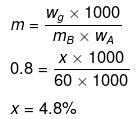Example 47. A very small amount of a non-volatile solute (that does not dissociate) is dissolved in 56.8 cm3 of benzene (density 0.889 g cm-3). At room temperature, vapour pressure of this solution is 98.8 mm Hg while that of benzene is 100 mm Hg. Find the molality of the solution. If the freezing temperature of this solution is 0.73 degree lower than that of benzene, what is the value of molal freezing point depression constant of benzene?
Solution.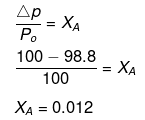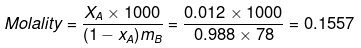ΔTf = Kf × Molality
0.73 = Kf × 0.1557
Kf = 4.688

Example 48. x g of a non-electrolytic compound (molar mass = 200) is dissolved in 1.0 litre of 0.05 M NaCl solution. The osmotic pressure of this solution is found to be 4.92 atm at 27ºC. Calculate the value of `x'. Assume complete dissociation of NaCl and ideal behaviour of this solution.
(a) 16.52 gm
(b) 24.032 gm
(c) 19.959 gm
(d) 12.35 gm
Ans. (c)
Solution.
(i) For NaCl : p = iCRT = 2 × 0.05 × 0.0821 × 300 = 2.463 atm
(ii) For unknown compound,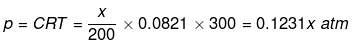Total osmotic pressure p = p+ p2
4.92 = 2.463 + 0.1231 x
x = 19.959 g

Example 49. The freezing point of a solution containing 50 cm3 of ethylene glycol in 50 g of water is found to be -34ºC. Assuming ideal behaviour, calculate the density of ethylene glycol (Kf for water = 1.86 K kg mol-1).
(a) 1.13 g/cm3
(b) 2.00 g/cm3
(c) 1.8 g/cm3
(d) 2.25 g/cm3
Ans. (a)
Solution.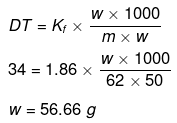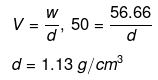Example 50. Match the boiling point with Kb for x, y and z if molecular weight of x, y and z are same.

 b. pt Kb x 100 0.68 y 27 0.53 z 253 0.98

Solution. Molal elevation constant may be calculated as,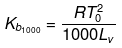(where, Tºb = boiling point of pure solvent Lv = latent heat of vaporization.)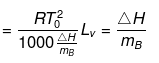(here, ΔHV = molar latent heat of vaporization).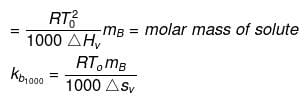here, ΔSV = entropy of vaprization.
By considering ΔSV as almost constant, Kb µ Tº.
Kb(x) = 0.68 ; Kb (y) = 0.53 and Kb (z) = 0.98.

Example 51. 1.22 g C6H5COOH is added into two solvents and data of ΔTb and Kb are given as -
(a) ln 100 g CH3COCH3; ΔTb= 0.17; Kb = 1.7 kg kelvin/mol
(b) ln 100 g benzene; ΔTb = 0.13; Kb = 2.6 kg kelvin/mol
Find out the molecular weight of C6H5COOH in both cases and inerpret the result.
Solution.
(a)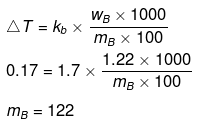(b)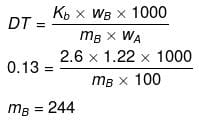(Abnormally double molecular mass of benzoic acid, it shows association of benzoic acid in benzene).

Example 52. How much C2H5OH should be added to 1 litre H2O so that it will not freeze at -20ºC?
Kf = 1.86ºC/m
Solution. Mass of 1 litre water = 1000 g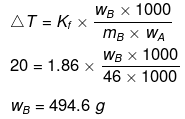Example 53. Calculate the molarity of each of the ions in solution when 3.0 litre of 4.0 M NaCl and 4.0 litre of 2.0 M CoCl2 are mixed and diluted to 10 litre.
Solution. Molarity Na  = 1.2 M
Molarity Co2+  = 0.8 M
Molarity Cl- = 2.8 M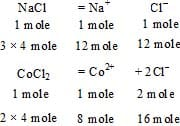Total Cl- ions = 28 mole.

Example 54. Calculate the molarity of each ion in solution after 2.0 litre of 3.0 M AgNO3 is mixed with 3.0 litre of 1.0 M BaCl2.
Solution.
BaCl2+  2AgNO→ 2AgCl +Ba(NO3)2
Initial 3 6 -_
mole
Final - -6 (ppt) 3
mole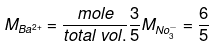Example 55. 1.2 kg ethylene glycol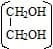was added in a car radiator containing 9 litre water. The freezing of water was just prevented when car was running in the Himalayan valley at temperature -4ºC. Sudden thunderstorm in the valley lowered the temperature to -6ºC. Calculate the amount of ice separated.
Solution.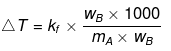A → Solute; B → Solvent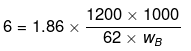wB = 6000 g
weight of ice = (Total weight of H2O) - (wt. of H2O at 6°C) = 9000 - 6000 = 3000 g = 3 kg

Example 56. The vapour pressure of a solvent decreased by 10 mm of mercury when a non-volatile solute was added to the solvent. The mole fraction of the solute in the solution is 0.2. What should be the mole fraction of the solvent, if the decrease in the vapour pressure is to be 20 mm of mercury.
(a) 0.8
(b) 0.6
(c) 0.4
(d) 0.2
Ans. (b)
Solution.
Mole fraction of solute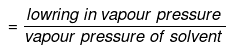Comparing under the two conditions,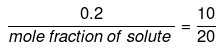or mole fraction of solute = 0.4
mole fraction of solvent = (1 -0.4) = 0.6

Example 57. Three solutions of HCl having normality 12 N, 6 N and 2 N are mixed to obtain a solutions of 4 N normality. Which among the following volume ratio is correct for the above three components?
(a) 1 : 1 : 5
(b) 1 : 2 : 6
(c) 2 : 1 : 9
(d) 1 : 2 : 4
Ans. (b)
Solution.
Use Hit & Trial Method.
N1V+ N2V+ N3V3 = NR(V+ V+ V3)
12 × 1 6 × 2 2 × 6 = NR(9)
NR = 4

Example 58. Two solutions of H2SO4 of molarities x and y are mixed in the ratio of V1 mL : V2 mL to form a solution of molarity M1. If they are mixed in the ratio of V2mL : V1mL, they form a solution of molarity M2. Given V1. Given V1/V2 => 1 and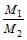=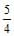, then x : y is -
(a) 2 : 1
(b) 4 : 1
(c) 1 : 2
(d) 3 : 1
Ans. (a)
Solution.
Molarity of the mixture can be calculated as.
M1V+ M2V2 = MR(V+ V2)
where, M= resultant solution
(V1 × x) × (V2 × y) = M1(V+ V2)
(V2 × x) × (V1 × y) = M2(V+ V2)
Dividing equation (i) by equation (ii), we get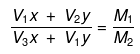Substituting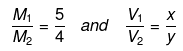we can calculate x : y.

Example 59. Isuling (C2H10O5)n is dissolved in a suitable solvent and the osmotic pressure (p) of solutions of various concentrations (g/cc) C is measured at 20ºc. The slope of the plot of p against 'C' is found to be 4.65 × 10-3. The molecular weight of insulin is -
(a) 4.8 × 105
(b) 9 × 105
(c) 3 × 105
(d) 5.17 × 106
Ans. (d)
Solution.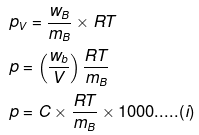where C = concentration in g/cc,
Comparing eqs. (i) and (ii), ...(ii)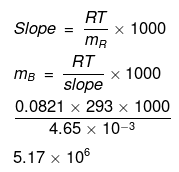Example 60. Compound PdCl4.6H2O is a hydrated complex; 1 molal aqueous solution of it has freezing point 269.28 K. Assuming 100% ionization of complex, calculate the molecular formula of the complex (Kf for water = 1.86 K kg mol-1)
(a) [Pd(H2O)6]Cl4
(b) [Pd(H2O)4Cl2]Cl 2.2H2O
(c) [Pd(H2O)3Cl3]Cl.3H 2O
(d) [Pd(H2O)2Cl4].4H 2O
Ans. (c)
Solution.
ΔT = i × K× m
(273 -269.28) = i × 1.86 × 1
3.72 = i × 1.86
i = 2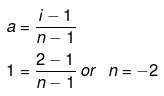Thus, the complex should give two ions in the solution, i.e., the complex will be [Pd(H2O)3Cl3]Cl.3H2O]

Example 61. pH of 0.1 M monobasic acid is measured to be 2. Its osmotic pressure at a given temperature T K is -
(a) 0.1 RT
(b) 0.11 RT
(c) 1.1 RT
(d) 0.01 RT
Ans. (b)
Solution.
HA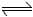H+  A-
t = 0  C  0     0
teq C -C∝ C∝ C∝
[H ] = Ca, [H ] = 10-pH
Cα = 10-2
0.1 α = 10-2
a = 0.1
a =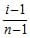; 0.1 =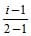i = 1.1
p = iCRT
= 1.1 × 0.1 × RT = 0.11 RT

Example 62. Lowering of vapour pressure in 1 molal aqueous solution at 100ºC is -
(a) 13.44 mm Hg
(b) 14.12 mm Hg
(c) 31.2 mm Hg
(d) 35.2 mm Hg
Ans. (a)
Solution.
Molality and mole fraction are related as follows: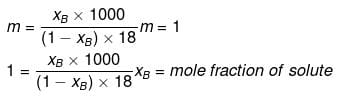mA = molar mass of solvent
xB = 0.0176, x= 0.9824
p = p°AxA
p = 760 × 0.9824 = 746.624
Δp = p°A - p = 760 - 746.624 = 13.4 mm Hg.

The document Solved Examples - Solutions (Part - 4) Notes | Study Additional Documents and Tests for JEE - JEE is a part of the JEE Course Additional Documents and Tests for JEE.
All you need of JEE at this link: JEE

## Additional Documents and Tests for JEE

11 videos|159 docs|14 tests
 Use Code STAYHOME200 and get INR 200 additional OFF

## Additional Documents and Tests for JEE

11 videos|159 docs|14 tests

Track your progress, build streaks, highlight & save important lessons and more!

,

,

,

,

,

,

,

,

,

,

,

,

,

,

,

,

,

,

,

,

,

;